Скачать презентацию Chp 5 Little Book Motion Math Work

2f58d5cc723a93661907ce5d27cf1461.ppt

• Количество слайдов: 45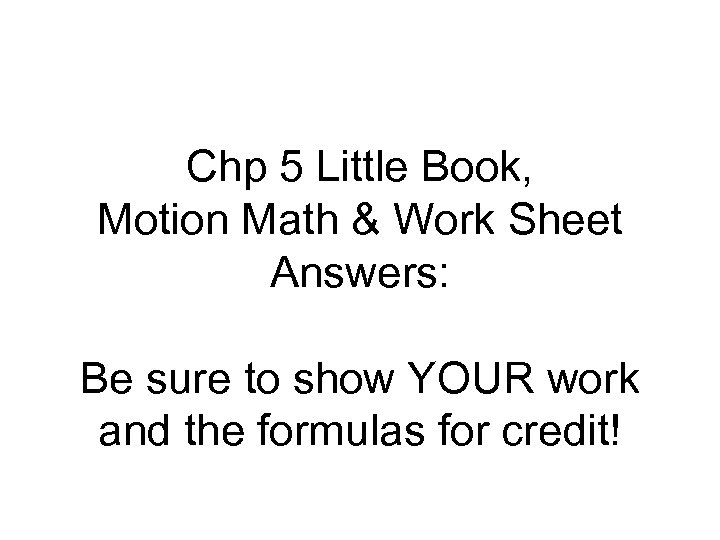Chp 5 Little Book, Motion Math & Work Sheet Answers: Be sure to show YOUR work and the formulas for credit!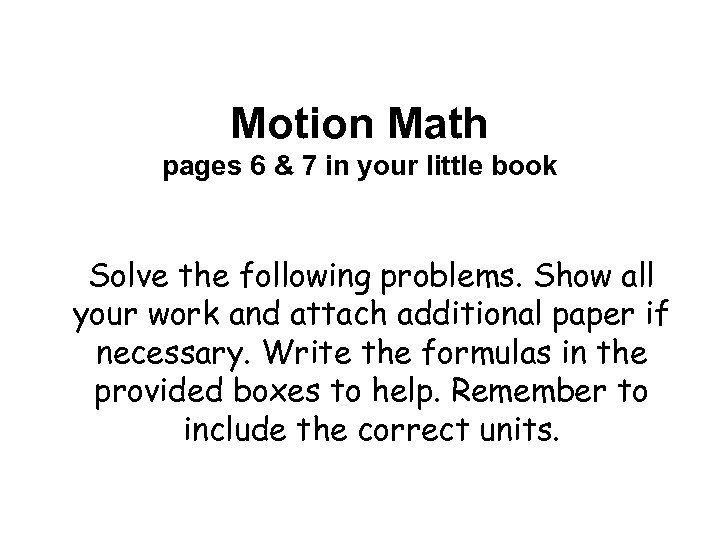Motion Math pages 6 & 7 in your little book Solve the following problems. Show all your work and attach additional paper if necessary. Write the formulas in the provided boxes to help. Remember to include the correct units.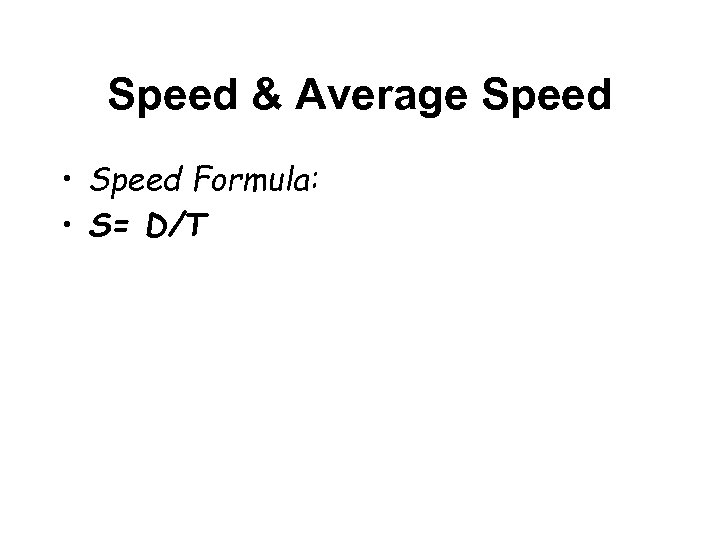Speed & Average Speed • Speed Formula: • S= D/T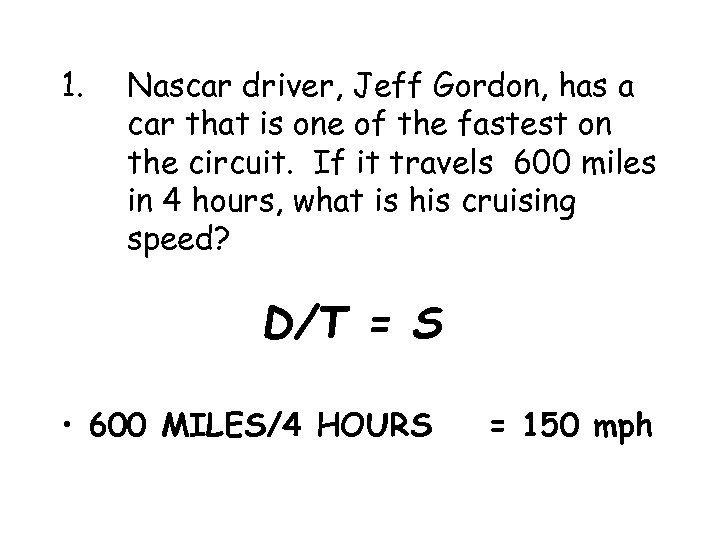1. Nascar driver, Jeff Gordon, has a car that is one of the fastest on the circuit. If it travels 600 miles in 4 hours, what is his cruising speed? D/T = S • 600 MILES/4 HOURS = 150 mph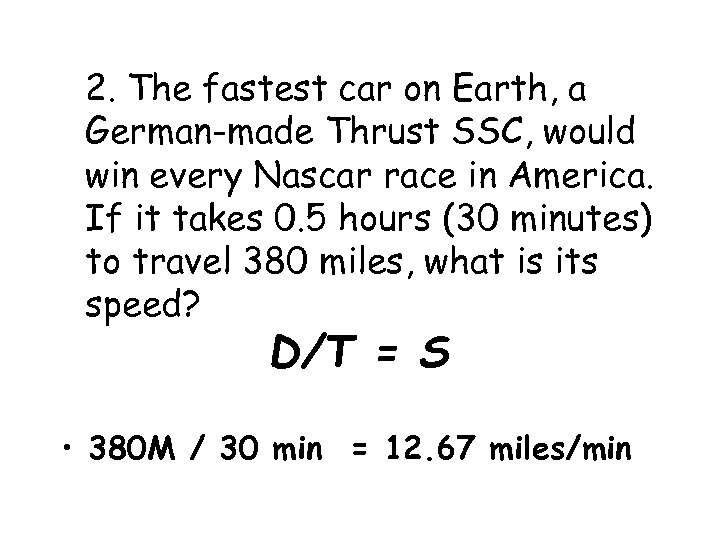2. The fastest car on Earth, a German-made Thrust SSC, would win every Nascar race in America. If it takes 0. 5 hours (30 minutes) to travel 380 miles, what is its speed? D/T = S • 380 M / 30 min = 12. 67 miles/min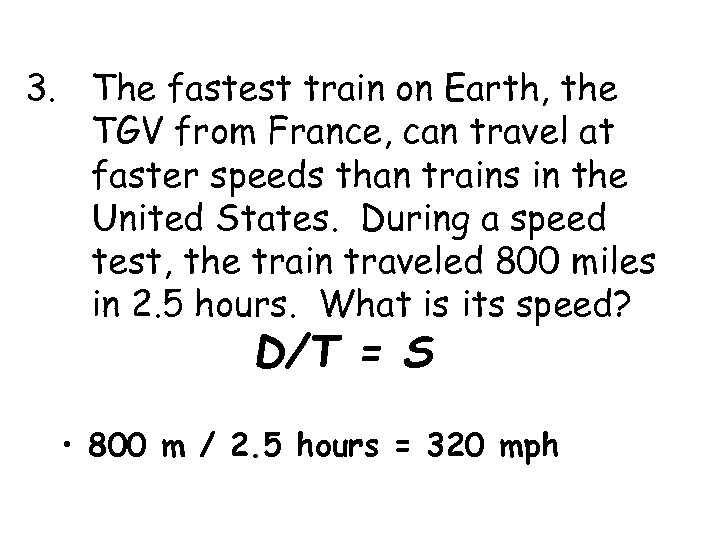3. The fastest train on Earth, the TGV from France, can travel at faster speeds than trains in the United States. During a speed test, the train traveled 800 miles in 2. 5 hours. What is its speed? D/T = S • 800 m / 2. 5 hours = 320 mph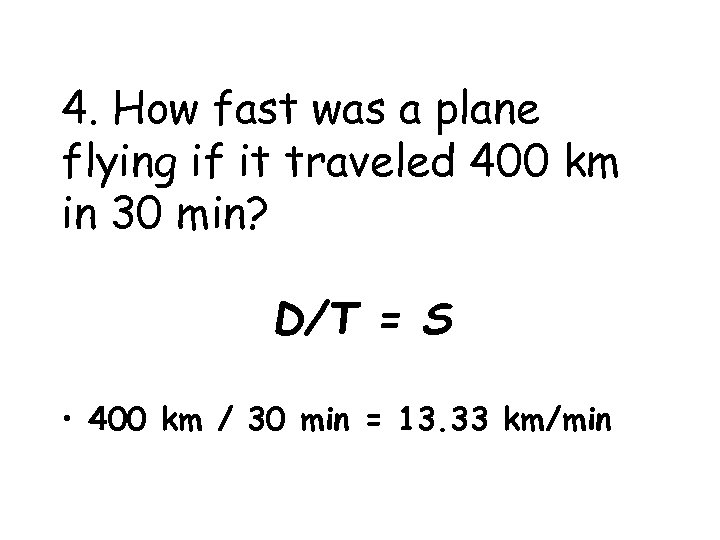4. How fast was a plane flying if it traveled 400 km in 30 min? D/T = S • 400 km / 30 min = 13. 33 km/min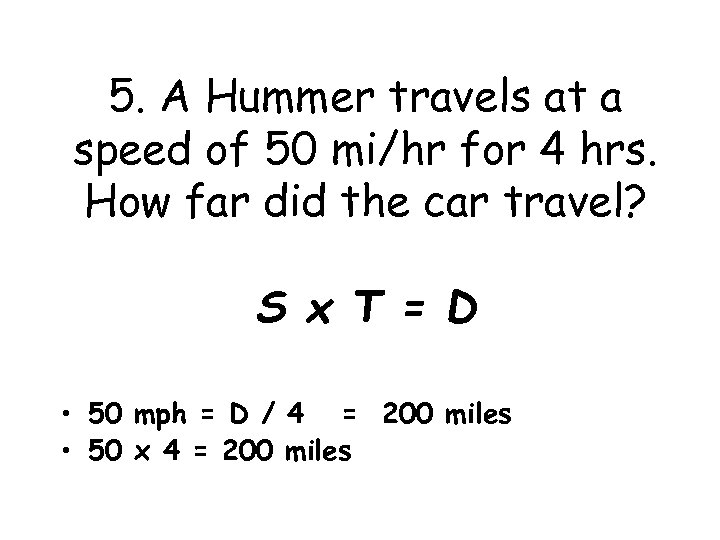5. A Hummer travels at a speed of 50 mi/hr for 4 hrs. How far did the car travel? S x T = D • 50 mph = D / 4 = 200 miles • 50 x 4 = 200 miles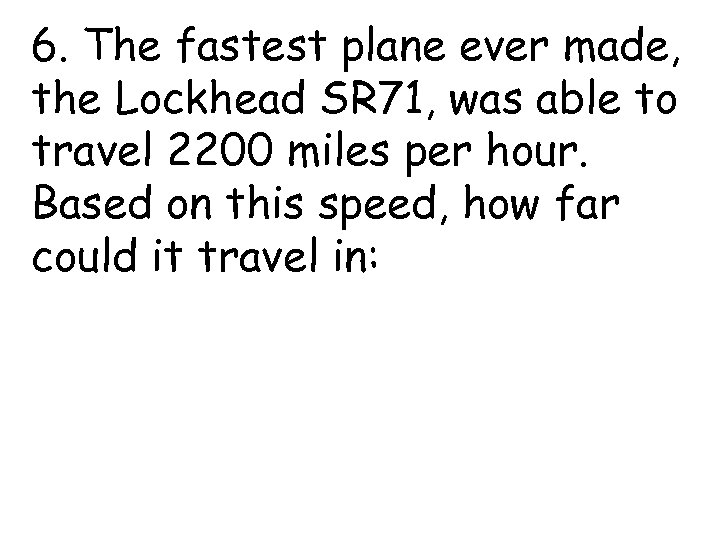6. The fastest plane ever made, the Lockhead SR 71, was able to travel 2200 miles per hour. Based on this speed, how far could it travel in: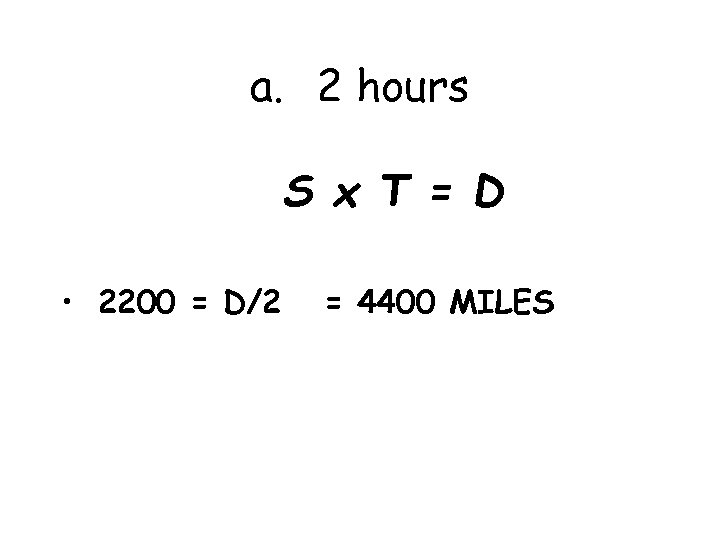a. 2 hours S x T = D • 2200 = D/2 = 4400 MILES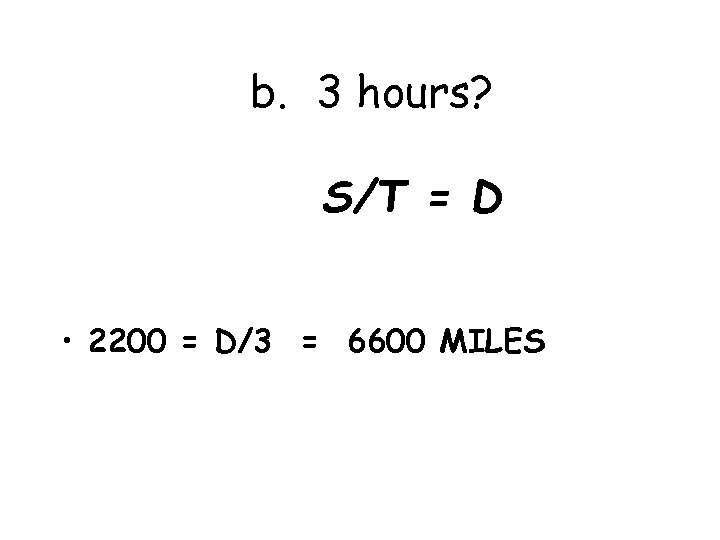b. 3 hours? S/T = D • 2200 = D/3 = 6600 MILES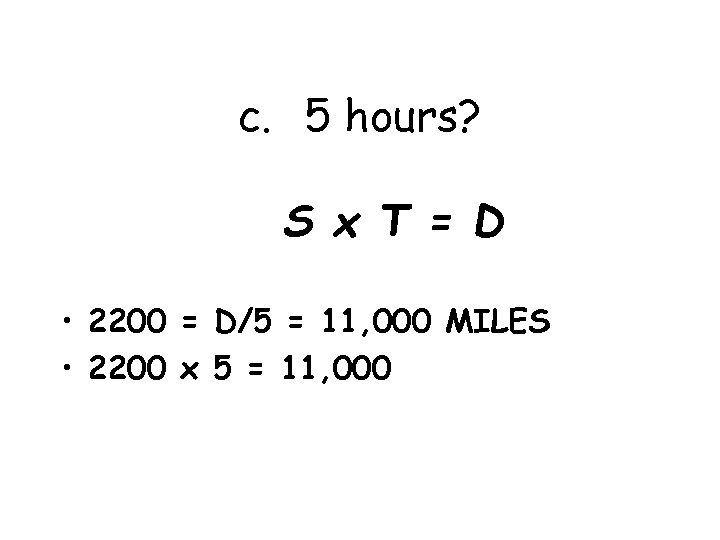c. 5 hours? S x T = D • 2200 = D/5 = 11, 000 MILES • 2200 x 5 = 11, 000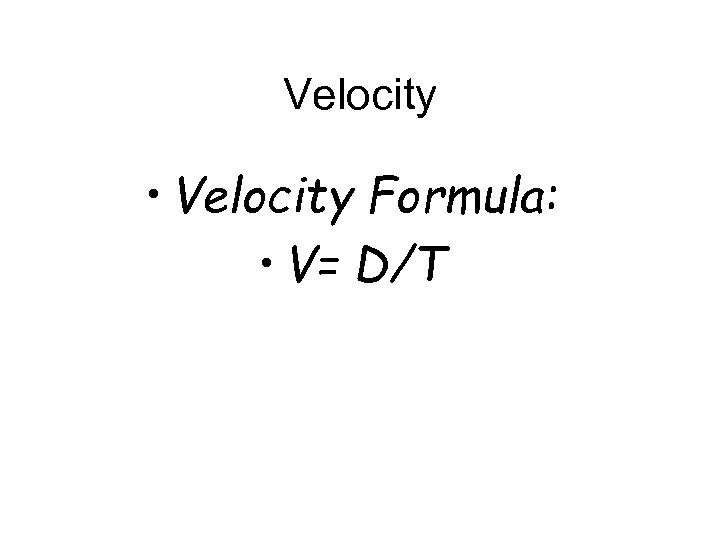Velocity • Velocity Formula: • V= D/T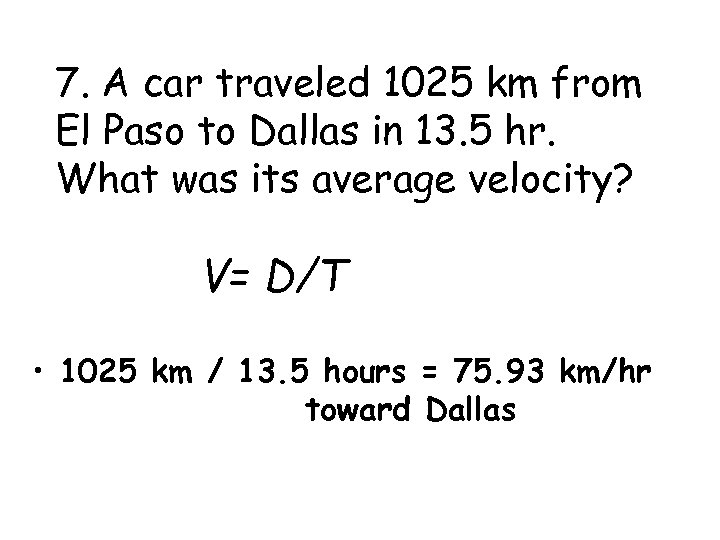7. A car traveled 1025 km from El Paso to Dallas in 13. 5 hr. What was its average velocity? V= D/T • 1025 km / 13. 5 hours = 75. 93 km/hr toward Dallas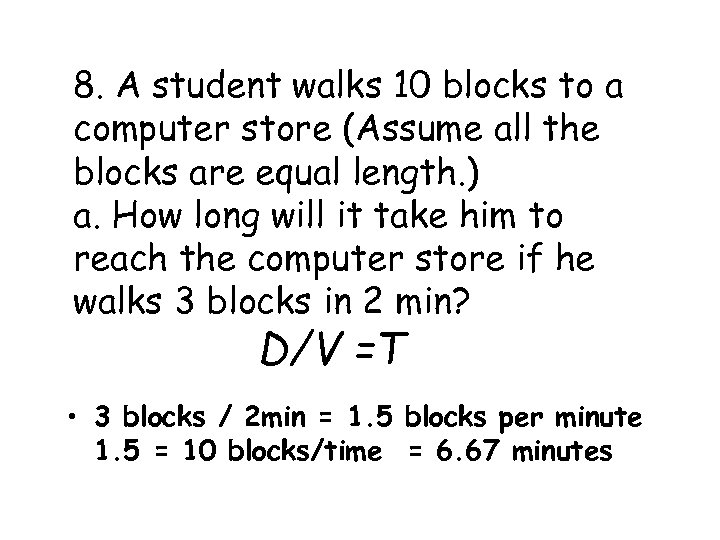8. A student walks 10 blocks to a computer store (Assume all the blocks are equal length. ) a. How long will it take him to reach the computer store if he walks 3 blocks in 2 min? D/V =T • 3 blocks / 2 min = 1. 5 blocks per minute 1. 5 = 10 blocks/time = 6. 67 minutes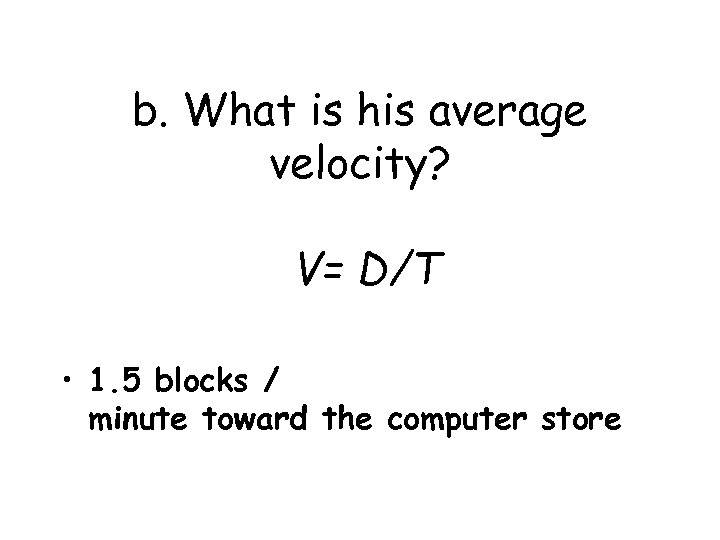b. What is his average velocity? V= D/T • 1. 5 blocks / minute toward the computer store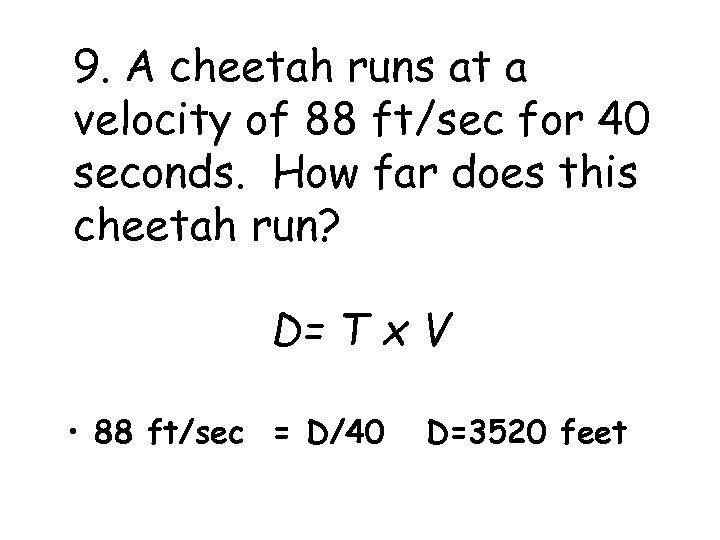9. A cheetah runs at a velocity of 88 ft/sec for 40 seconds. How far does this cheetah run? D= T x V • 88 ft/sec = D/40 D=3520 feet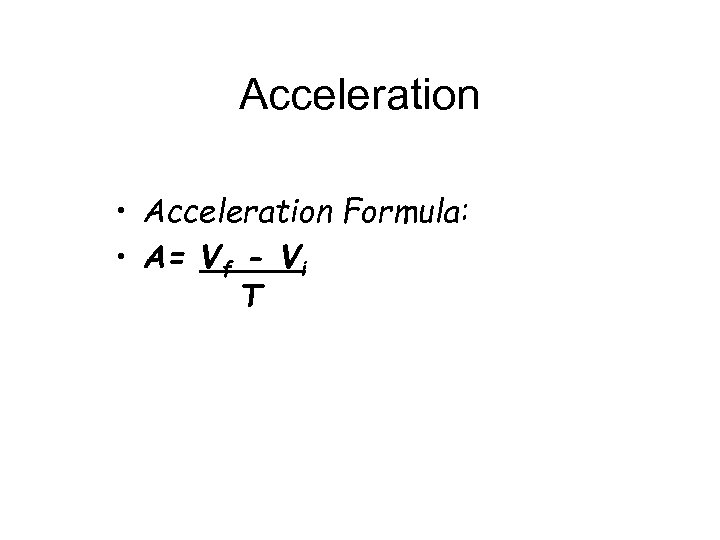Acceleration • Acceleration Formula: • A= Vf - Vi T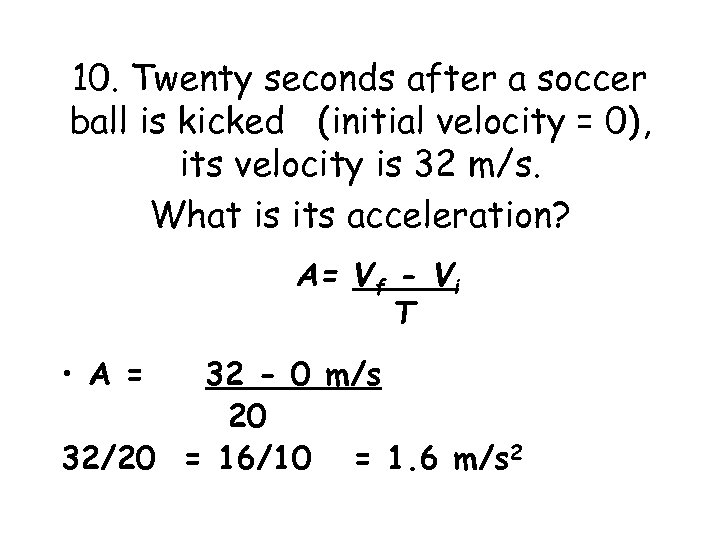10. Twenty seconds after a soccer ball is kicked (initial velocity = 0), its velocity is 32 m/s. What is its acceleration? A= Vf - Vi T • A = 32 - 0 m/s 20 32/20 = 16/10 = 1. 6 m/s 2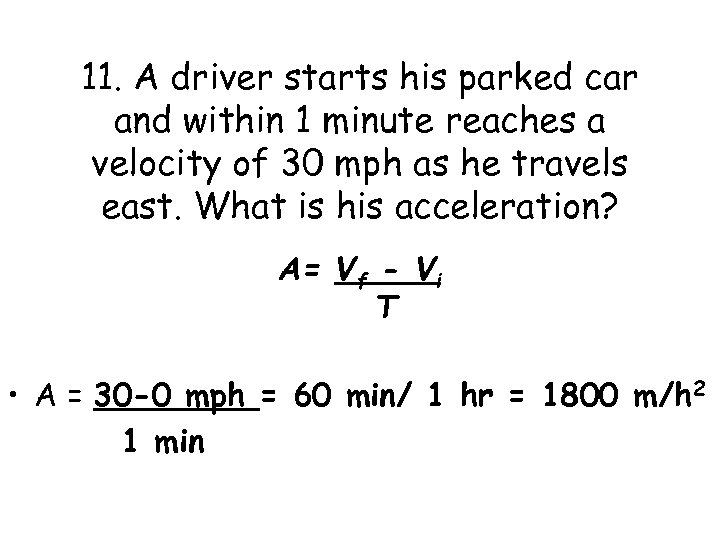11. A driver starts his parked car and within 1 minute reaches a velocity of 30 mph as he travels east. What is his acceleration? A= Vf - Vi T • A = 30 -0 mph = 60 min/ 1 hr = 1800 m/h 2 1 minRandom – Find the missing variable Page 7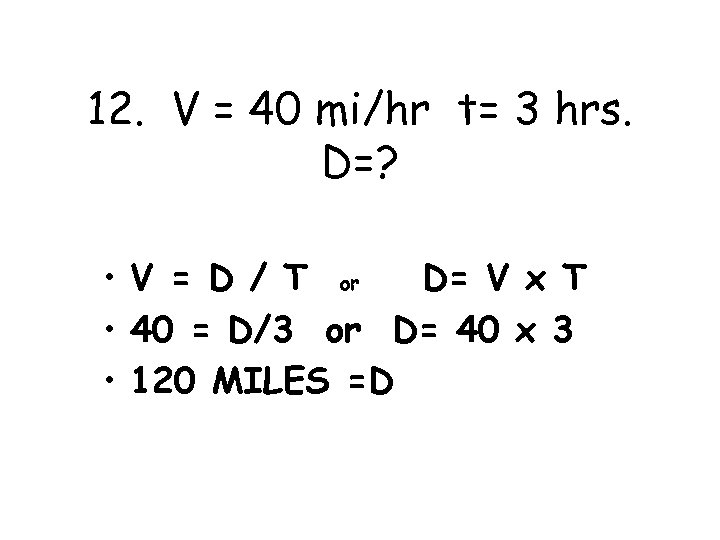12. V = 40 mi/hr t= 3 hrs. D=? • V = D / T or D= V x T • 40 = D/3 or D= 40 x 3 • 120 MILES =D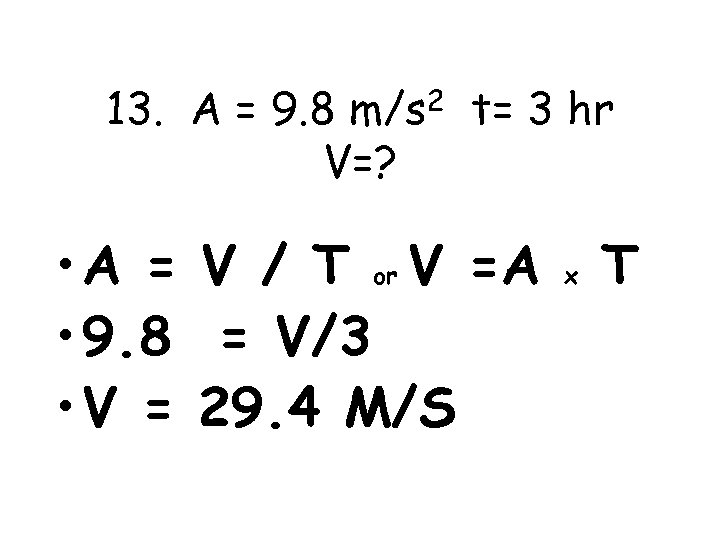13. A = 9. 8 m/s 2 t= 3 hr V=? • A = V / T or V =A • 9. 8 = V/3 • V = 29. 4 M/S x T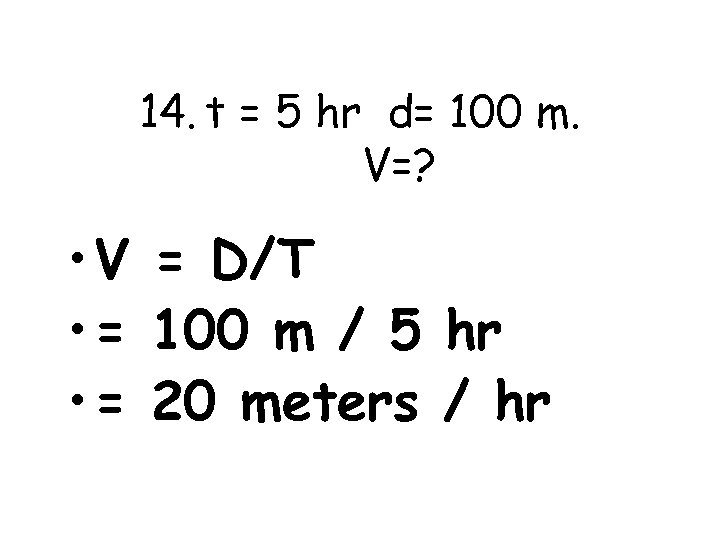14. t = 5 hr d= 100 m. V=? • V = D/T • = 100 m / 5 hr • = 20 meters / hr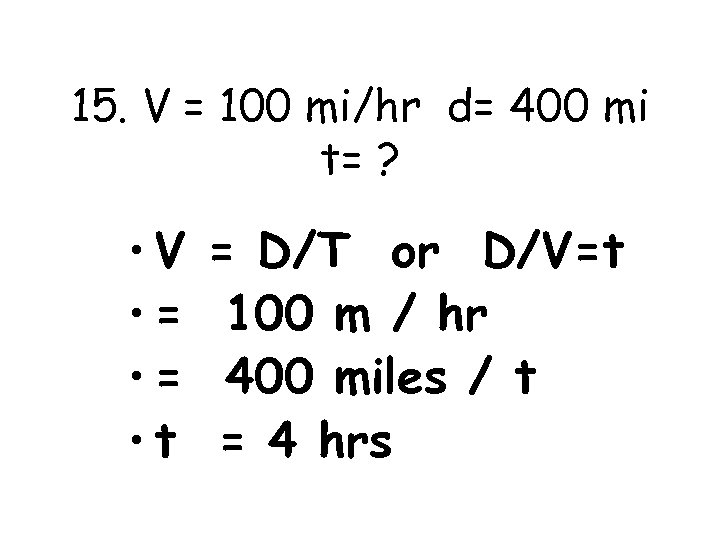15. V = 100 mi/hr d= 400 mi t= ? • V • = • t = D/T or D/V=t 100 m / hr 400 miles / t = 4 hrs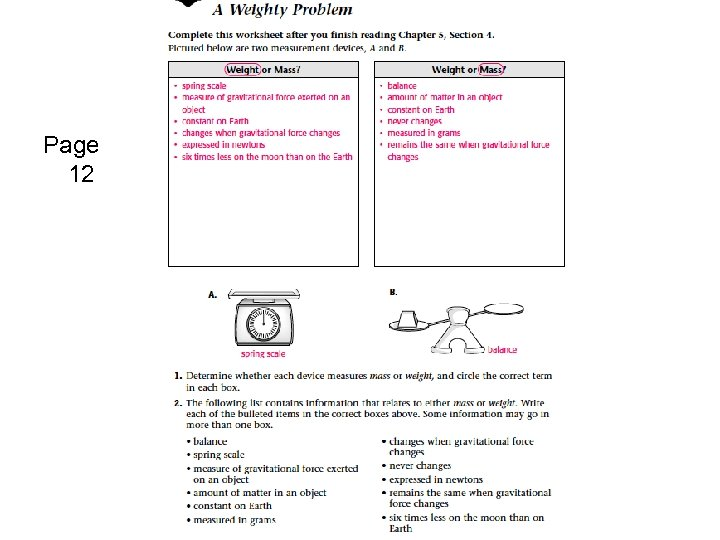Page 12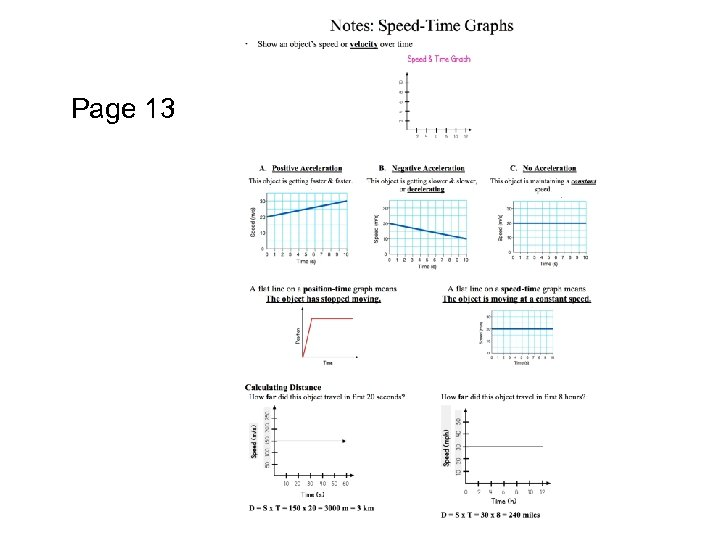Page 13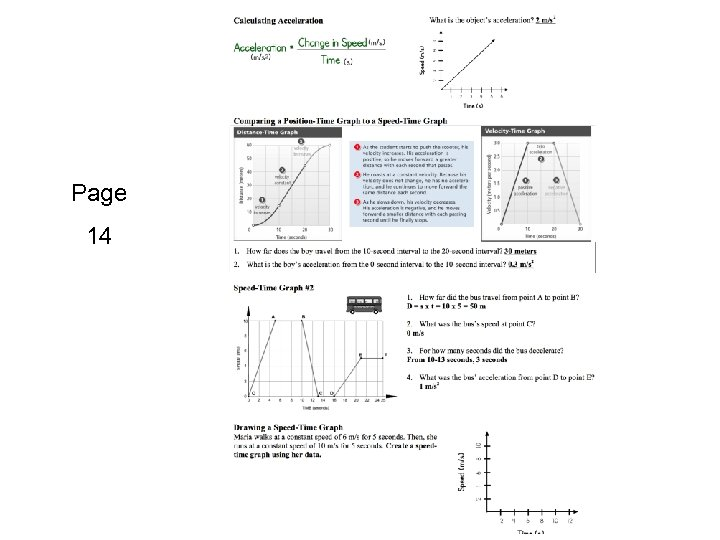Page 14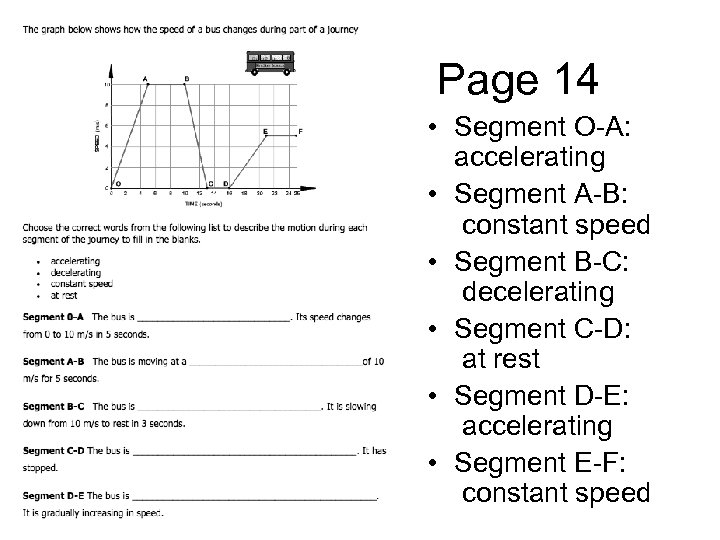Page 14 • Segment O-A: accelerating • Segment A-B: constant speed • Segment B-C: decelerating • Segment C-D: at rest • Segment D-E: accelerating • Segment E-F: constant speed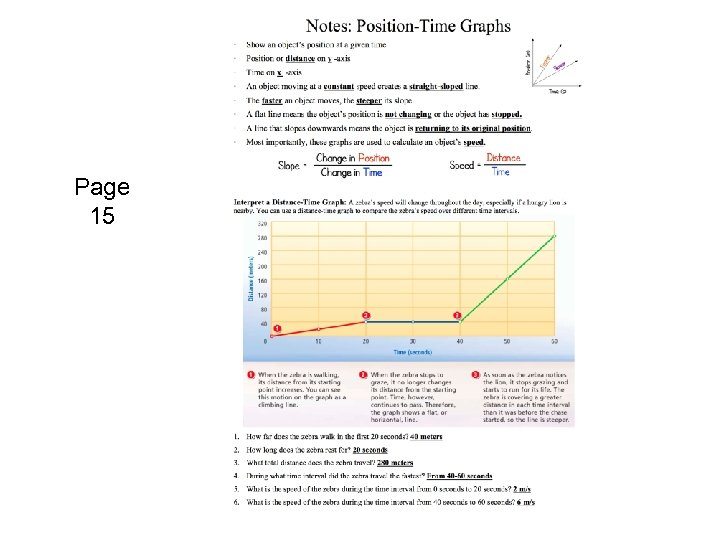Page 15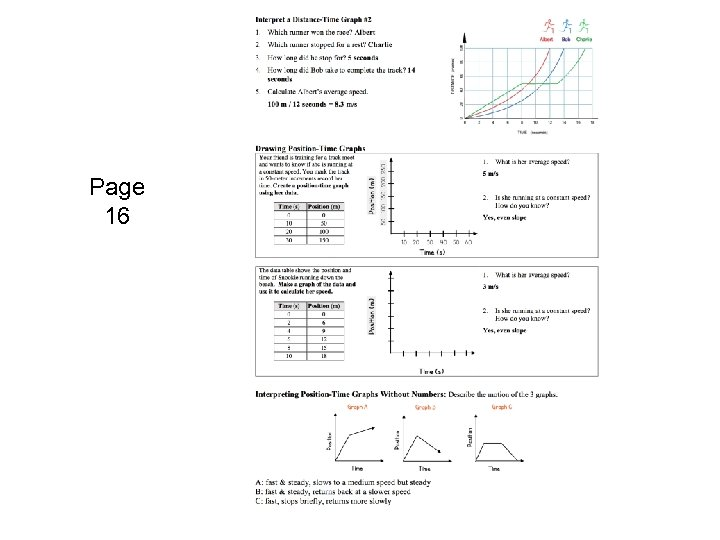Page 16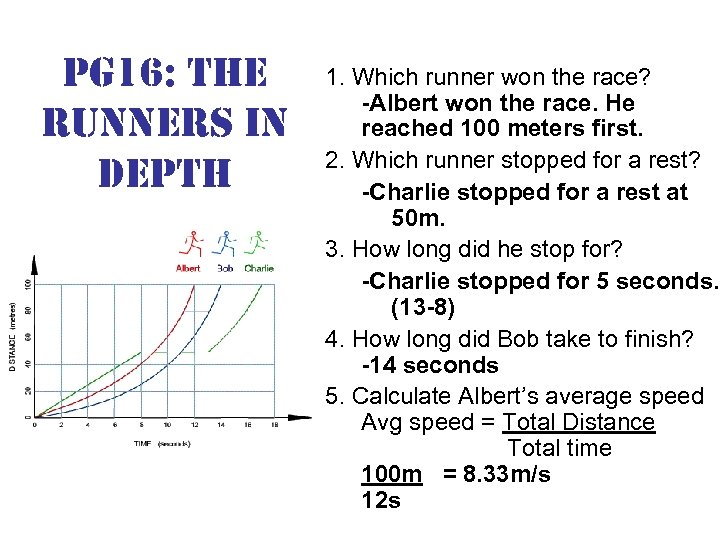pg 16: the runners in depth 1. Which runner won the race? -Albert won the race. He reached 100 meters first. 2. Which runner stopped for a rest? -Charlie stopped for a rest at 50 m. 3. How long did he stop for? -Charlie stopped for 5 seconds. (13 -8) 4. How long did Bob take to finish? -14 seconds 5. Calculate Albert’s average speed Avg speed = Total Distance Total time 100 m = 8. 33 m/s 12 sMotion Graphs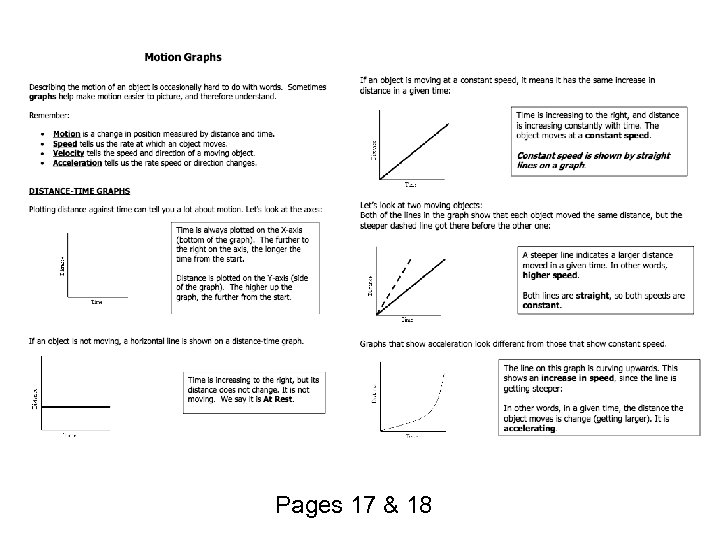Pages 17 & 18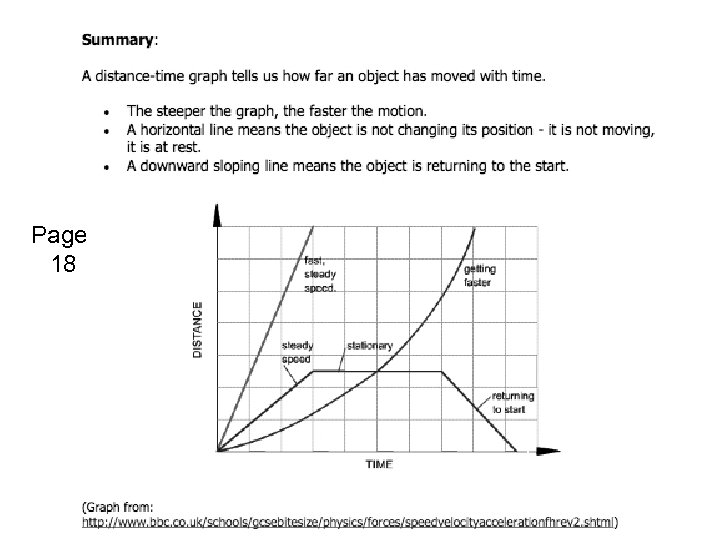Page 18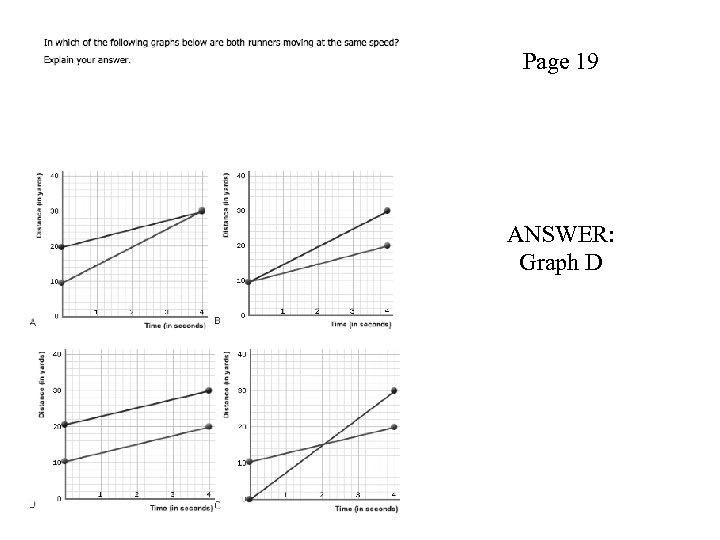Page 19 ANSWER: Graph D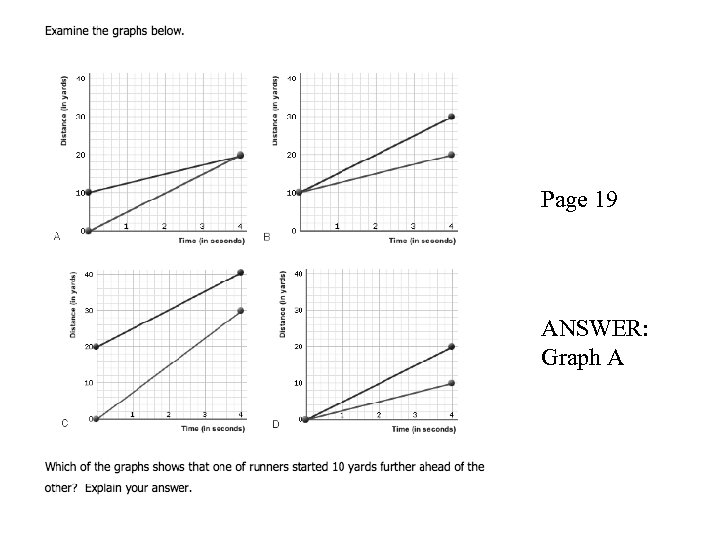Page 19 ANSWER: Graph A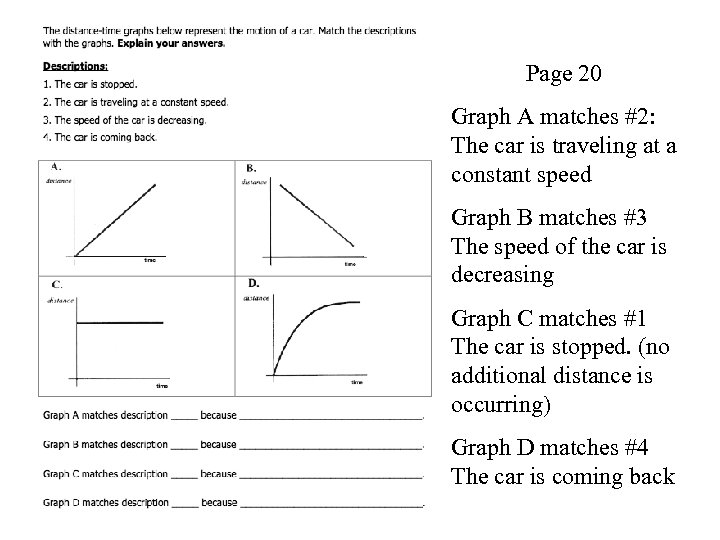Page 20 Graph A matches #2: The car is traveling at a constant speed Graph B matches #3 The speed of the car is decreasing Graph C matches #1 The car is stopped. (no additional distance is occurring) Graph D matches #4 The car is coming back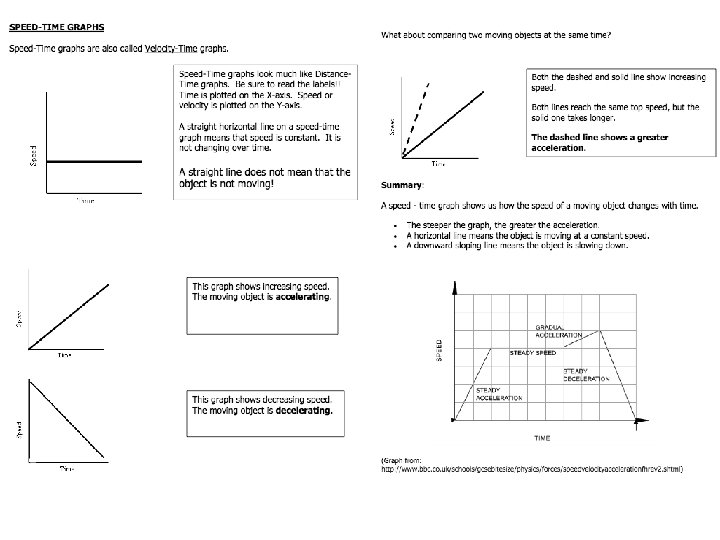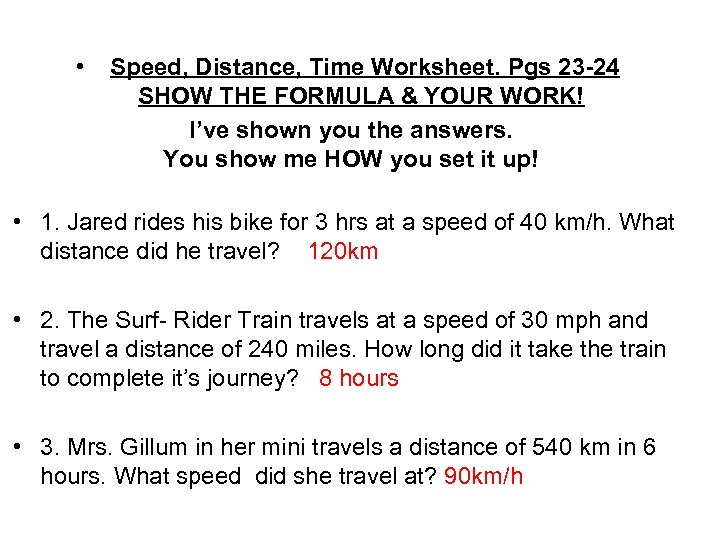• Speed, Distance, Time Worksheet. Pgs 23 -24 SHOW THE FORMULA & YOUR WORK! I’ve shown you the answers. You show me HOW you set it up! • 1. Jared rides his bike for 3 hrs at a speed of 40 km/h. What distance did he travel? 120 km • 2. The Surf- Rider Train travels at a speed of 30 mph and travel a distance of 240 miles. How long did it take the train to complete it’s journey? 8 hours • 3. Mrs. Gillum in her mini travels a distance of 540 km in 6 hours. What speed did she travel at? 90 km/h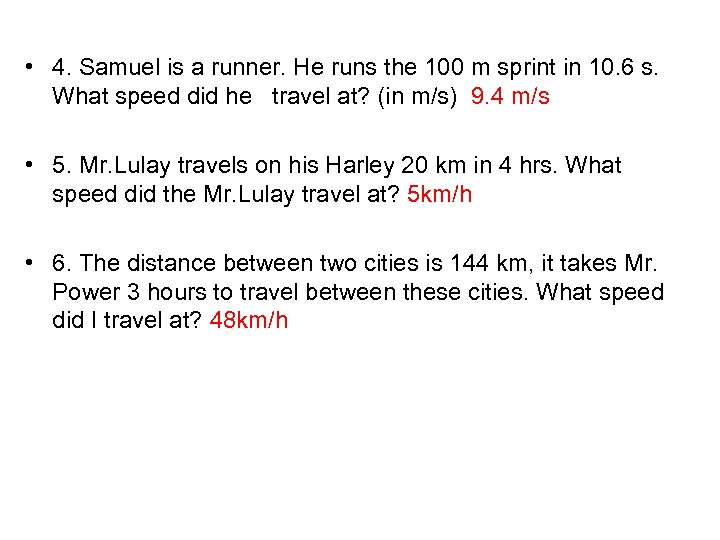• 4. Samuel is a runner. He runs the 100 m sprint in 10. 6 s. What speed did he travel at? (in m/s) 9. 4 m/s • 5. Mr. Lulay travels on his Harley 20 km in 4 hrs. What speed did the Mr. Lulay travel at? 5 km/h • 6. The distance between two cities is 144 km, it takes Mr. Power 3 hours to travel between these cities. What speed did I travel at? 48 km/h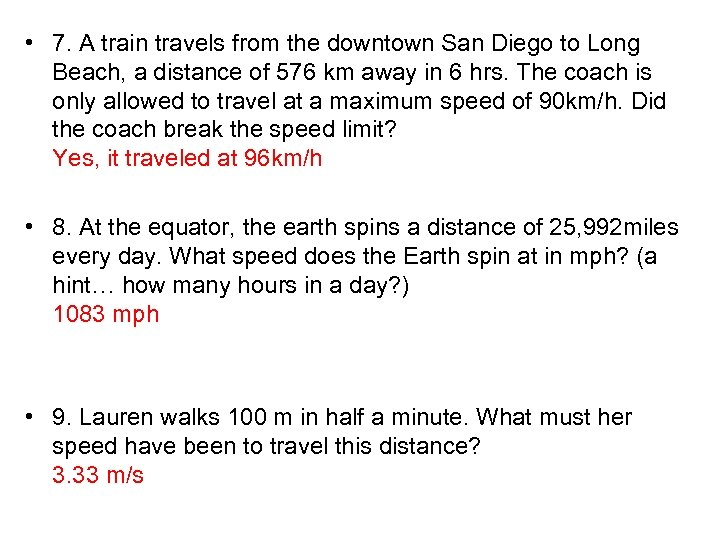• 7. A train travels from the downtown San Diego to Long Beach, a distance of 576 km away in 6 hrs. The coach is only allowed to travel at a maximum speed of 90 km/h. Did the coach break the speed limit? Yes, it traveled at 96 km/h • 8. At the equator, the earth spins a distance of 25, 992 miles every day. What speed does the Earth spin at in mph? (a hint… how many hours in a day? ) 1083 mph • 9. Lauren walks 100 m in half a minute. What must her speed have been to travel this distance? 3. 33 m/s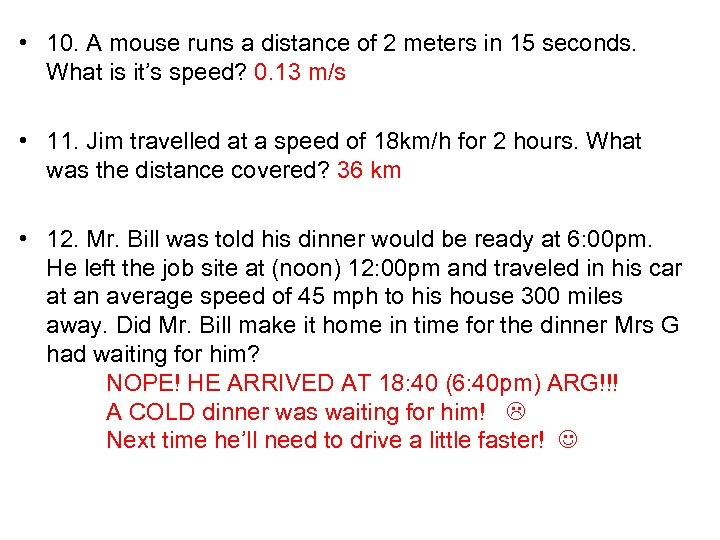• 10. A mouse runs a distance of 2 meters in 15 seconds. What is it’s speed? 0. 13 m/s • 11. Jim travelled at a speed of 18 km/h for 2 hours. What was the distance covered? 36 km • 12. Mr. Bill was told his dinner would be ready at 6: 00 pm. He left the job site at (noon) 12: 00 pm and traveled in his car at an average speed of 45 mph to his house 300 miles away. Did Mr. Bill make it home in time for the dinner Mrs G had waiting for him? NOPE! HE ARRIVED AT 18: 40 (6: 40 pm) ARG!!! A COLD dinner was waiting for him! Next time he’ll need to drive a little faster!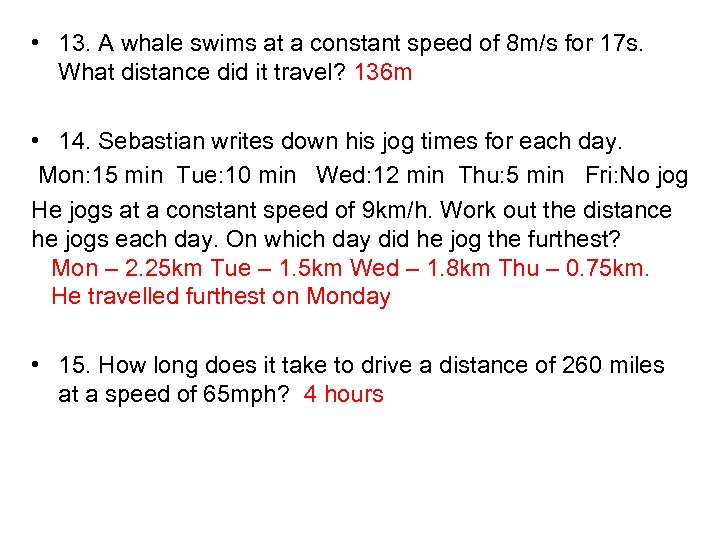• 13. A whale swims at a constant speed of 8 m/s for 17 s. What distance did it travel? 136 m • 14. Sebastian writes down his jog times for each day. Mon: 15 min Tue: 10 min Wed: 12 min Thu: 5 min Fri: No jog He jogs at a constant speed of 9 km/h. Work out the distance he jogs each day. On which day did he jog the furthest? Mon – 2. 25 km Tue – 1. 5 km Wed – 1. 8 km Thu – 0. 75 km. He travelled furthest on Monday • 15. How long does it take to drive a distance of 260 miles at a speed of 65 mph? 4 hours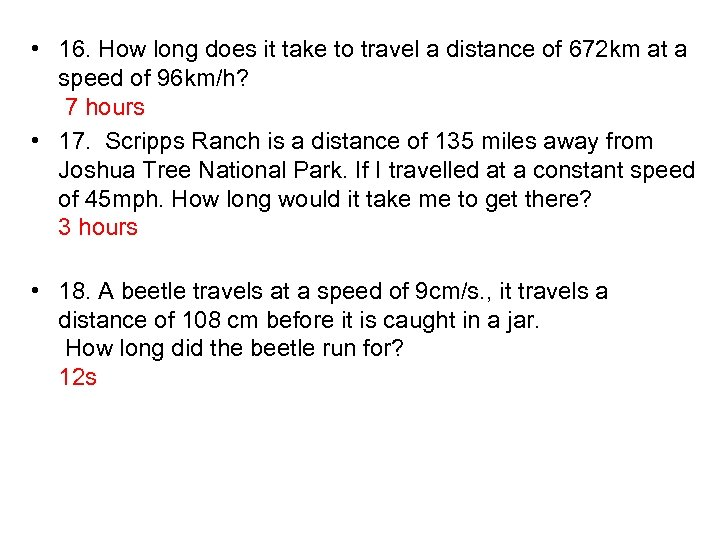• 16. How long does it take to travel a distance of 672 km at a speed of 96 km/h? 7 hours • 17. Scripps Ranch is a distance of 135 miles away from Joshua Tree National Park. If I travelled at a constant speed of 45 mph. How long would it take me to get there? 3 hours • 18. A beetle travels at a speed of 9 cm/s. , it travels a distance of 108 cm before it is caught in a jar. How long did the beetle run for? 12 s